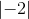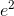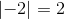SAT II Math II : Mathematical Relationships

Example Questions

Example Question #71 : Sat Subject Test In Math Ii

Solve the absolute value: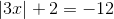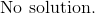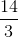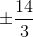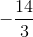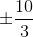Explanation:

Subtract 2 from both sides.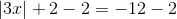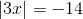Under this condition, there are no values ofthat will give a negative 14.

The answer is:Example Question #72 : Sat Subject Test In Math Ii

Solve: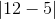Explanation:

The lines on the outside of this problem indicate it is an absolute value problem. When solving with absolute value, remember that it is a measure of displacement from 0, meaning the answer will always be positive.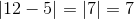For this problem, that gives us a final answer of 7.

Example Question #73 : Sat Subject Test In Math Ii

Solve: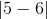Explanation:

The lines on the outside of this problem indicate it is an absolute value problem. When solving with absolute value, remember that it is a measure of displacement from 0, meaning the answer will always be positive.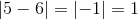For this problem, that gives us a final answer of 1.

Example Question #11 : Absolute Value

Solve: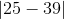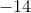Explanation:

The lines on the outside of this problem indicate it is an absolute value problem. When solving with absolute value, remember that it is a measure of displacement from 0, meaning the answer will always be positive.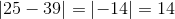For this problem, that gives us a final answer of 14.

Example Question #81 : Sat Subject Test In Math Ii

Solve: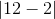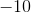Explanation:

The lines on the outside of this problem indicate it is an absolute value problem. When solving with absolute value, remember that it is a measure of displacement from 0, meaning the answer will always be positive.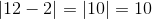For this problem, that gives us a final answer of 10.

Example Question #82 : Sat Subject Test In Math Ii

Solve: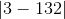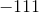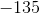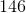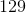Explanation:

The lines on the outside of this problem indicate it is an absolute value problem. When solving with absolute value, remember that it is a measure of displacement from 0, meaning the answer will always be positive.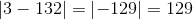For this problem, that gives us a final answer of 129.

Example Question #21 : Absolute Value

Solve: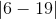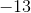Explanation:

The lines on the outside of this problem indicate it is an absolute value problem. When solving with absolute value, remember that it is a measure of displacement from 0, meaning the answer will always be positive.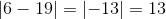For this problem, that gives us a final answer of 13.

Example Question #22 : Absolute Value

Solve: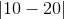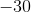Explanation:

The lines on the outside of this problem indicate it is an absolute value problem. When solving with absolute value, remember that it is a measure of displacement from 0, meaning the answer will always be positive.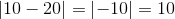For this problem, that gives us a final answer of 10.

Example Question #23 : Absolute Value

Solve: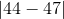Explanation:

The lines on the outside of this problem indicate it is an absolute value problem. When solving with absolute value, remember that it is a measure of displacement from 0, meaning the answer will always be positive.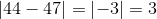For this problem, that gives us a final answer of 3.

Example Question #24 : Absolute Value

Solve: